Next: 5. Conclusions Up: Multigrid Improvements to CITCOM Previous: 3. Multigrids   Contents

Subsections

4. Refinement

This chapter covers two topics of interest as suggested in the project proposal:
1. Prolongation and Restriction
These operations may help improve the rate of convergence.
2. Local Mesh Refinement
For example a better convergence rate may be achieved by refining part of the mesh in regions with high velocity gradients.

Each of these topics is discussed below.

4.1 Prolongation and Restriction

For the last phase (phase 3) of the project, two work packages are defined to implement: (i) local mesh refinement and; (ii) improvements to prolongation and restriction operations by way of implementing arithmetic, geometric and harmonic averaging and matrix-dependent transfer. The topic of prolongation and restriction required investigation before it could be implemented in the existing scenario so that it is of benefit to CITCOM. Prolongation and restriction operations help update the nodal values for the nodes at upper and lower levels in the multigrid context with nodal values from lower and upper levels respectively. An appropriate implementation of these operations, by adopting different averaging schemes  is expected to help improve the rate of convergence.

In this context, the study of current implementations for restriction (based on weighted averaging) and prolongation (based on weighted interpolation) along with the previous experience and discussion with PI on this topic helped in reaching the conclusion that it is unlikely to gain any significant advantage by implementing suggested averaging schemes over the existing implementation. Therefore, it was agreed (with PI and NAG) that any implementation of prolongation and restriction operations as suggested in the proposal may not be of any advantage. Following on from this decision, the remaining time of nearly four weeks for this current phase was allocated to local mesh refinement. The initial part of it had been spent studying the current implementation, and on exploring ways to help the implementation as suggested in the original proposal.

4.2 Local Mesh Refinement

Following on from the basic concepts introduced in section 3.7.1, the intention here is to extend the concept in the context of the adopted scheme for implementation within CITCOM as detailed below.

4.2.1 Refinement Strategy

One way to express the challenge of local mesh refinement may be: to increase the spatial resolution of some parts of the domain or sub-domain which are dynamically selected following some specific criteria. Of course the choice of these parts is not obvious, nevertheless, for the general description of the method, we have assumed that a refinement criteria is available and the refinement area is known before hand. In this particular case, this refinement area is a certain fraction of the z-dimension covering at least two elements or an even number of elements if there are more than two elements in the base level (level 0) mesh. To avoid the load imbalance situation and to capture most of the sharp gradients e.g. for velocity, which occur near the earth's surface where the lithosphere is located, all elements in x- and y-dimension are refined. This yields the lower part of the mesh as unrefined or coarse and the upper part of the mesh (near and at the earth's surface) as refined at base level. Once this local refinement of the mesh near the top of domain is achieved at base level, which now consists of elements of two different size: (i) coarse elements and (ii) refined elements achieved by bisecting a coarse element in to two sub elements in each dimension yielding four refined elements in two dimension and eight refined elements in three dimension, all of the mesh is refined for the higher level meshes in the nested mesh hierarchy. For more information on this point a revisit to the sub section 2.2.1 would be of help to understand the way the Cartesian coordinate system is used within CITCOM.

Due to the fact that no specific local mesh refinement strategy for implementation is identified in the proposal. We have discussed the pointers to the ways it can be achieved to a certain depth, detail and a number of possible schemes for local mesh refinement in the context of the regular structured mesh elements used in CITCOM. To conclude this we have decided to implement the one-level-difference refinement rule as it fulfils the requirement of only one level of refinement at the top of the domain without triggering the load imbalance problem.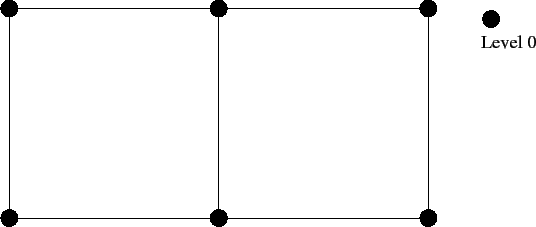Here we describe the one-level-difference refinement rule based on local mesh refinement in more detail. In order to illustrate the local mesh refinement we have used, we shall show refinement of squares/rectangles in two dimension and cubes/cuboids in three dimensions. Figure 4.1 consists of a two element mesh in two dimensions and shall be used to explain the local mesh refinement where all mesh elements and nodes are at level 0.Let us assume that we wish to refine this mesh up to two levels with refinement at and near the right-bottom corner of the mesh. This implies that any element near the right-bottom corner of the mesh at level 0 is a candidate for refinement and the resulting new elements from the refinement of that element form the mesh at level 1. Subsequently, any element at level 1 at or near the right-bottom corner is refined again and the new elements are constructed to make up the mesh at level 2. This scenario is depicted in figure 4.2 for elements in two dimensions where refined or sub elements are obtained by bisecting a coarse level element in each dimension, in this example along the x- and y-dimensions, to generate four new elements each time an element is refined. After two levels of refinement of the elements near the right-bottom corner, we get a total of eight elements; (i) one element at level 0, (ii) three elements at level 1, and (iii) four elements at level 2. The corresponding nodes at level 0 are marked with a black dot, at level 1 with a small circle and at level 2 with a larger circle. Any node shared by two elements which are at different levels are marked by a black dot and a small circle or a small and a large circle accordingly. However we note that this refinement strategy does not obey the one-level-difference refinement rule.

From the point of view of refinement although it is perfectly acceptable but in the given multigrid context, it is not workable. It makes the mapping between different mesh levels hard and it becomes harder and harder (if not impossible) as the mesh is further refined to higher levels. Therefore, it is not the most suitable refinement scheme for CITCOM.Another possible scenario is to refine the element at or near the right-bottom corner to the desired refinement level, which is level 2 in this case, as shown in figure 4.3. This creates elements which are at the two extremes in the mesh; (i) the largest elements at coarse level and (ii) elements which are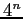times smaller in two dimensions and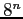times smaller in three dimensions than the coarse level elements where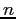is the highest level of refinement. This refinement scheme is forbidden by the one-level-difference refinement rule. Additionally, this creates a larger number of hanging nodes on the edge(s) of coarser elements, this is an undesired situation as handling any hanging nodes not only requires extra work but is hard to deal with too.

Let us again assume that we wish to refine the same two element mesh up to two levels with refinement at or near the right-bottom corner of the mesh. However, this time we obey the one-level-difference refinement rule, that is, any two elements in the fully refined mesh (up to level 2 in this case) are at levels which are immediately next to each other in the nested hierarchy of the refined meshes. In other words, no element is at level 0 when this two element mesh is refined to level 2 as shown in figure 4.4. This shows that elements in the mesh after refinement are at the difference of a maximum of one level from any other element in the mesh. Therefore, to refine the elements at or near the right-bottom corner of the mesh to level 2, other elements in the mesh, which would have not been refined otherwise, must be refined at least up to level 1. This is an additional restriction to the one-level-difference refinement rule described in  and . This ensures that all elements and nodes in the mesh are either at level 1 or level 2 avoiding the complex situation of multilevel nesting of mesh elements which becomes very hard to handle if not impossible in multigrid prolongation and restriction operations.We note that in order to achieve the mesh refinement shown in figure 4.4, all elements at level 0 must be refined when an element at or near the right-bottom corner is refined a second time. Elements whose nodes are marked with blue circles represent those elements which are refined as a consequence of refinement of other elements in the mesh. This can easily be generalised so that all elements at level n-1 must be refined when any element at level n is refined so that all elements in the resulting mesh are either at level n or n+1.

Having described the strategy for local mesh refinement by following the one-level-difference refinement rule, we setup some principals and restrictions in the context of the current implementation in CITCOM. It is assumed that:

• Only part of the mesh along the z-axis, in two and three dimension, is refined. Recall that Cartesian coordinates in CITCOM are defined as (x,z,y) in contrast to the customary definition as (x,y,z).

• Part of the mesh which is refined has elements at one level higher than the level of elements which are not refined.

• After local refinement of the mesh at level 0, base level coarse elements and new elements resulting from refinement are considered at level 0. Refinement to the next higher level is carried out throughout the mesh to maintain the similar mesh structure at each level.

• A node on a coarse element edge which is at the interface with refined elements is dealt by the refined or higher level elements only. For the coarse element, any such node is treated as non existent.

• Each mesh level consists of elements of two sizes; coarser elements and refined elements although both at the same level.

• A node on a coarse element edge which is shared by refined elements (at least two or more) is accounted for the refined elements only. Therefore, the overall mesh is considered as having no hanging nodes.

4.2.2 Refinement Setup

Following on from the strategy described in section 4.2.1, here we describe the local mesh refinement implemented in CITCOM, with the help of an example mesh in two dimensions. To start with, we have chosen a basic mesh of a reasonably small size in two dimensions with eight elements in the x-dimension and six elements in the z-dimension. It is distributed into four sub-domains as shown by the dark lines in figure 4.5.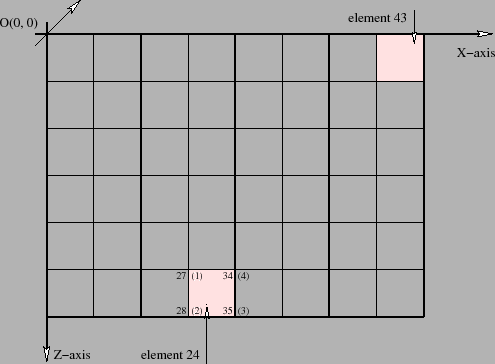In order to solve each sub-domain problem, we employ one process per sub-domain so that four processes are employed to solve the global problem; four along the x-dimension and one along the z-dimension, that is, each process covers the whole length along z-axis. Note that CITCOM does not allow more than one process in the z-dimension. As a by product, this helps in keeping the load of all processes reasonably balanced and no communication is required in the z-direction. This is also one of the reasons for choosing this one-level-difference refinement rule. The connectivity for a representative finite element numbered as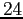is given by node numbers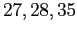and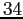corresponding to the local nodes numbersand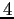respectively. This also reveals that element numbering in the mesh starts from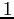near the origin (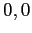) and is incremented in the z-direction followed by the x-direction. Similarly, (global) node numbering follows the same pattern whereas local numbering is anti-clockwise. This is extended to include the y-axis as a third dimension in the case of three dimensions as shown in figure 4.6. For the sake of clarity this is for a relatively smaller mesh size.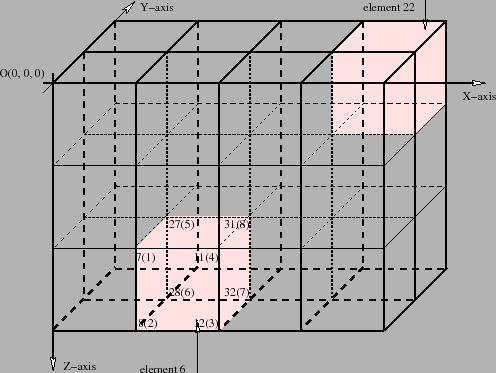Following the one-level-difference refinement rule and assumptions described in section 4.2.1, the mesh shown in figure 4.5 is refined at the top of the domain covering two elements in the z-direction, all eight elements in the x-direction and all two elements in the y-direction. This local refinement replaces existing elements with the new elements which are half the size and twice the number of the original elements in all dimensions. In other words, each refined element is replaced by four smaller elements of one-fourth the size of the original element in two dimensions as shown in figure 4.7 and by eight smaller elements of one-eighth the size of original element in three dimensions as shown in 4.8 (for a relatively smaller mesh size for the sake of clarity) respectively.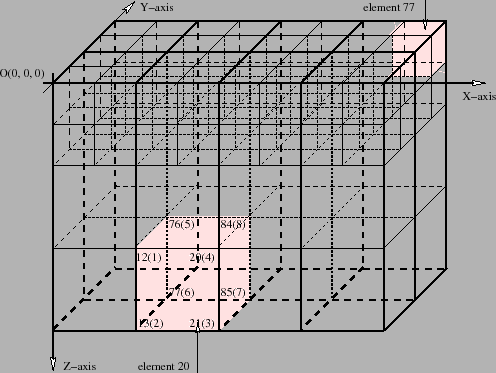However, it is important to note that these new elements are at the same level as the parent elements, which is the base level or level 0. After the setup of this base level mesh following the local mesh refinement, every next level in the nested mesh hierarchy is obtained by refining all elements in the mesh so that at any given mesh level, elements at the top of the domain are always smaller than the other elements in the mesh at that level but all elements are at the same level.

In order to specify the refinement area at the base level mesh, a bounding box is defined in the CITCOM input file and consists of two or three pairs of values, namely, (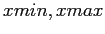), () and (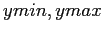) in two dimensions or three dimensions respectively. As these values are defined in the input file, the mesh refinement area is controlled at run time which offers the extra flexibility of changing the refinement area for experimentation without recompiling the whole source code.

The implementation of local mesh refinement in CITCOM required a major rewrite of a large number of functions, particularly related to the setup of C structures defined in CITCOM which provides the backbone to the functionality and construction of elements, sub elements, nodes, surface nodes, neighbouring nodes, node maps, coordinates, domain decomposition, neighbouring sub domains, communication routes, boundary conditions, setup for prolongation and restriction operations, etc. A brief description of a couple of these structures, their setup and how to access these structure members, modifications to the code, etc. are described in the following section.

4.2.3 Refinement Implementation

In this section, we try to reflect on the C structures defined in CITCOM source code by using snapshots of just two out of a total of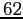structures. These are in addition to a large number of macros and look-up tables which are used to construct and fill-up these and other structures and arrays used for a variety of computational tasks. For example, populating of IEN struct with global node numbers for a given mesh level and element number with the help of a look-up table for the local node numbers of an element and then using it to populate the NEI struct with the number of elements surrounding any given node and mesh level and so on. One of these structures is All_variables, the top level structure is given below. It shows that a large number of other structures are nested in it along with other variables. The size of these nested structures vary ranging from having a few to a large number of member variables.

struct All_variables
struct All_variables {
struct TRACERS            tracers;
struct HAVE               Have;
struct BAVE               Bulkave;
struct TOTAL              Total;
struct MESH_DATA          mesh;
struct MESH_DATA          lmesh;
struct CONTROL            control;
struct OUTPUT             output;
struct MONITOR            monitor;
struct DATA               data;
struct SLICE              slice;
struct Segment            segment;
struct Slabs              slabs;
struct Crust              crust;
struct Parallel           parallel;
struct INFO               info;
struct Shape_function     N;
struct Shape_function_dx  Nx;
struct Shape_function1    M;
struct Shape_function1_dx Mx;
struct Shape_function1    L;
struct Shape_function1_dx Lx;
struct NEI                NEI[MAX_LEVELS];
struct COORD              *eco;
struct IEN                *ien;
struct SIEN               *sien;
struct ID                 *id;
struct LM                 *lmd;
struct LM                 *lm;
struct Shape_function_dx  *gNX;
struct Shape_function_dA  *gDA;
struct COORD              *ECO[MAX_LEVELS];
struct IEN                *IEN[MAX_LEVELS];
struct ID                 *ID[MAX_LEVELS];
struct SUBEL              *EL[MAX_LEVELS];
struct EG                 *elt_del[MAX_LEVELS];
struct EK                 *elt_k[MAX_LEVELS];
struct FNODE              *TWW[MAX_LEVELS];
struct LM                 *LMD[MAX_LEVELS];
struct Shape_function_dx  *GNX[MAX_LEVELS];
struct Shape_function_dA  *GDA[MAX_LEVELS];

FILE                      *fp;
FILE                      *filed;

higher_precision          *Eqn_k1[MAX_LEVELS];
higher_precision          *Eqn_k2[MAX_LEVELS];
higher_precision          *Eqn_k3[MAX_LEVELS];

int                       *surf_node;
int                       *surf_element;
int                       *mat;
int                       *Node_map[MAX_LEVELS];
int                       *Node_eqn[MAX_LEVELS];
int                       *Node_k_id[MAX_LEVELS];

unsigned int              *node;
unsigned int              *eqn;
unsigned int              *NODE[MAX_LEVELS];
unsigned int              *EQN[MAX_LEVELS];

float                     *stress;
float                     *Psi;
float                     *NP;
float                     *Mass;
float                     *tw;
float                     *Vi;
float                     *EVi;
float                     *Vielem;
float                     *T;
float                     *buoyancy;
float                     *Tdot;
float                     *EEDOTSQR;
float                     *dummy;
float                     *edummy;
float                     *C;
float                     *V;
float                     *V1;
float                     *Vest;
float                     *X;
float                     *XL;
float                     *VB;
float                     *TB;
float                     *edot;
float                     *MASS[MAX_LEVELS];
float                     *VI[MAX_LEVELS];
float                     *EVI[MAX_LEVELS];
float                     *TW[MAX_LEVELS];
float                     *XX[MAX_LEVELS];

double                    *P;
double                    *F;
double                    *H;
double                    *S;
double                    *U;
double                    *temp;
double                    *global_F;
double                    *dudx;
double                    *tau;
double                    *dtau;
double                    *spin;
double                    *BI[MAX_LEVELS];
double                    *BPI[MAX_LEVELS];
double                    **global_K;
double                    **factor_K;
};

In this All_variables structure, amongst others, struct Parallel is a nested structure at line 16 and consists of a few variables and other nested structures as its members as shown below.

struct Parallel
struct Parallel {
char                      machinename;

int                       me;
int                       nproc;
int                       nprocx;
int                       nprocz;
int                       nprocy;
int                       nprocxy;
int                       nproczy;
int                       nprocxz;
int                       automa;
int                       idb;
int                       log;
int                       num_b;
int                       me_loc;
int                       TNUM_PASS[MAX_LEVELS];
int                       START_ELE[MAX_LEVELS];
int                       START_NODE[MAX_LEVELS];
int                       *IDD[MAX_LEVELS];
int                       *ELE_ORDER[MAX_LEVELS];
int                       *NODE_ORDER[MAX_LEVELS];

struct                    BOUND  NUM_NNO[MAX_LEVELS];
struct                    BOUND  NUM_PASS[MAX_LEVELS];
struct                    BOUND  NUM_ELE[MAX_LEVELS];
struct                    PASS   NUM_NEQ[MAX_LEVELS];
struct                    PASS   NUM_NODE[MAX_LEVELS];
struct                    PASS   PROCESSOR[MAX_LEVELS];
struct                    BOUND  *NODE[MAX_LEVELS];
struct                    BOUND  *IDPASS[MAX_LEVELS];
struct                    PASS   *EXCHANGE_ID[MAX_LEVELS];
struct                    PASS   *EXCHANGE_NODE[MAX_LEVELS];
};

A code snapshot to find a (global) node number anywhere in the mesh prior to implementing local mesh refinement is given below. A very simple procedure is followed: loop over the number of nodes noy in the y-direction followed by a nested loop over the number of nodes nox in the x-direction and finally a third nested loop over the number of nodes noz in the z-direction which is not unusual in any such situation. At this point, (global) node number is determined using a simple formula given at line 4 of the code snapshot. This becomes even more simple in the two dimension case when looping over nodes in the y-direction has no affect and the first term on the right hand side of the equation at line 4 in the code snapshot vanishes. Looking at figure 4.5 in two dimension and figure 4.6 in three dimension, it is not hard to find a (global) node number for any given value of ix, jz and ky, the node numbers in the x-, z- and y-directions respectively.

Finding global node without local mesh refinement
for (ky=1; ky <= noy; ky++) {
for (ix=1; ix <= nox; ix++) {
for (jz=1; jz <= noz; jz++) {
node = (ky-1)*nox*noz + (ix-1)*noz + jz;
}/* end of for jz=1 */
}/* end of for ix=1 */
}/* end of for ky=1 */

Here the same is repeated for the local mesh refinement case. Loops over noy, nox and noz, nodes in the y-, x- and z-directions respectively are the same. However, we have to have additional checks in place to make sure that we are accounting for refined and coarser parts of the mesh accordingly. For this purpose we have defined extra variables (also members of struct INFO):

• znmax - maximum number of nodes in z-direction.
• znbnd - number of nodes in refined part of mesh (band) in z-direction.
• zxnd1 - number of nodes in zx-plan covering coarse and refined part of mesh.
• zxnd2 - number of nodes in zx-plan covering only refined part of mesh.

A few other local variables such as ynodes, xnodes, xnalt and znalt are also used. Looking at this code snapshot, figure 4.7 in two dimension and figure 4.8 in three dimension, it is clear that for these loops over noy, nox and noz, the number of nodes in the y-, x- and z-directions respectively, results in accumulating node numbers which are used to determine the (global) node number. The incremental contribution within each loop depends on these loop counters being odd, even, or some other combination of these.

Finding global node with local mesh refinement
int znmax = E->info.znmax;
int znbnd = E->info.znbnd;
int zxnd1 = E->info.zxnd1;
int zxnd2 = E->info.zxnd2;

ynodes = 0;
for (ky=1; ky <= noy; ky++) {
( 1==(ky%2) ) ? (xnalt=zxnd1) : (xnalt=zxnd2);
xnodes = 0;
for (ix=1; ix <= nox; ix++) {
if ( ky%2 ) {
( 1==(ix%2) ) ? (znalt=znmax) : (znalt=znbnd);
}
else {
znalt = znbnd;
}
for (jz=1; jz <= znalt; jz++) {
node = ynodes + xnodes + jz;
}/* end of for jz=1 */
xnodes += znalt;
}/* end of for ix=1 */
ynodes += xnalt;
}/* end of for ky=1 */

In the previous two paragraphs, with the help of code snapshots, we have compared how to determine a (global) node number before and after the local mesh refinement. Here we would compare the differences in setting up struct EL which determines sub elements for any given element and mesh level in the prior and post local mesh refinement context. In the former case, we have three nested loops (in three dimensions) over the number of elements ely, elx and elz in the y-, x- and z-directions respectively. The corresponding code snapshot is given below. Note that each element (being a parent) has two sub elements in each dimension: a total of four sub elements in two dimensions and eight elements in three dimensions. With the help of simple formulae, as before for the (global) node numbers, the number of each element and that of its first sub element is determined (see line number 11 and 12 in code snapshot). This and remaining sub elements are than fed to struct EL utilising a pre-defined look-up table and loop over sub elements. This procedure is repeated over all mesh levels, except the highest level, for all elements so that on completion we are in a position to access any sub element for any element at any mesh level except the top level.

Setup of struct EL prior to local mesh refinement
for (lev=E->mesh.levmax-1; lev >= E->mesh.levmin; lev--) {
elx = E->lmesh.ELX[lev];
elz = E->lmesh.ELZ[lev];
ely = E->lmesh.ELY[lev];
elxu = 2*elx;
elzu = 2*elz;

for (ye=1; ye <= ely; ye++) {
for (xe=1; xe <= elx; xe++) {
for (ze=1; ze <= elz; ze++) {
element = (ye-1)*elz*elx + (xe-1)*elz + ze;
sub_element = 2*(ye-1)*elzu*elxu + 2*(xe-1)*elzu + (2*ze - 1);

for (el=1; el <= ENODES[E->mesh.nsd]; el++) {
E->EL[lev][element].sub[el] = ( sub_element +
offset[el].vector[z] +
offset[el].vector[x]*elzu +
offset[el].vector[y]*elzu*elxu );
}/* end of for el=1 */
} /* end of for ye=1 */
} /* end of for ze=1 */
} /* end of for xe=1 */
}/* end of lev= ... */

The procedure to fill-up struct EL for sub elements in the post local mesh refinement scenario is not as simple as without local mesh refinement. The relevant code snapshot together with a brief dissection is given below.

In this case of local mesh refinement, we have, as previously, three nested loops (in three dimensions) over a number of elements ely, elx and elz in the y-, x- and z-directions respectively. We then determine the number of each element using a simple formula shown on line 24 of the code snapshot. In two dimensions, the loop over ely becomes ineffective. Note that the mesh elements in this case are of two different sizes: the refined part of the mesh consists of elements of one-fourth in size of elements in the coarse part of the mesh in two dimension and of one-eighth in size in three dimensions. The element number is determined for the mesh which is not yet refined. At this point, we need to differentiate between elements if they belong to the coarse or the refined part of the mesh and treat accordingly. This is determined with the help of a simple utility function. If an element belongs to refined part of the mesh, its sub elements or, more appropriately, corresponding refined elements which form the actual refined mesh are determined. These refined elements are at the same level (i.e. the current one) as those which belong to the coarse part of the mesh.

Before proceeding further, let us define some variables which are used in filling-up the struct EL.

• (xemin, zemin, yemin) - number of elements at current mesh level without refinement in x-, z- and y-directions respectively.

• (xebnd, zebnd) - number of elements at current mesh level in refined part of mesh (band) in x- and z-directions respectively.

• (xeminN, zeminN, yeminN) - number of elements at next upper level without refinement in x-, z- and y-directions respectively.

• (xebndN, zebndN) - number of elements at next upper level in refined part of mesh (band) in x- and z-directions respectively.

The variables defined at the current level determine the element numbers in the coarse and refined part of the mesh whereas the variables defined at next upper level determine sub elements corresponding to each element at the current level as long as the current level is not the highest level in the nested mesh hierarchy.

In the code snapshot, lines from 24 to 60 and from 65 to 90 show how sub elements corresponding to elements in the refined and coarse part of the mesh are determined and then feed to struct EL utilising a pre-defined look-up table and looping over sub elements respectively. This procedure, starts at the second highest level, it is then repeated over all mesh levels in a downward direction for all elements. On completion of this procedure, the setup of the struct EL is completed and sub elements of an element at any given mesh level except the top level become accessible.

Setup of struct EL with local mesh refinement
for (lev=E->mesh.levmax-1; lev >= E->mesh.levmin; lev--) {
xemin = E->info.ELXP[lev];
zemin = E->info.ELZP[lev];
yemin = E->info.ELYP[lev];

xebnd = E->info.XEBND[lev];
zebnd = E->info.ZEBND[lev];

next = lev+1;
xeminN = E->info.ELXP[next];
zeminN = E->info.ELZP[next];
yeminN = E->info.ELYP[next];

xebndN = E->info.XEBND[next];
zebndN = E->info.ZEBND[next];

for (ye=1; ye <= yemin; ye++) {
for (xe=1; xe <= xemin; xe++) {
for (ze=1; ze <= zemin; ze++) {
element = (ye-1)*zemin*xemin + (xe-1)*zemin + ze;

/* for refined element corresponding to base mesh */
if ( is_element_refined(E, lev, element) ) {
sub_element = 2*(ye-1)*zeminN*xeminN + 2*(xe-1)*zeminN + (2*ze-1);

for (elm=1; elm <= ends; elm++) {
element_count = ( element +
(ye-1)*(zebnd/2)*(xebnd/2)*(ends-1) +
(xe-1)*(zebnd/2)*(ends-1) + (ze-1) +
offset[elm].vector[z] +
offset[elm].vector[x]*zebnd +
offset[elm].vector[y]*zebnd*xebnd/xemin );

sub_element_count = ( sub_element +
offset[elm].vector[z] +
offset[elm].vector[x]*zeminN +
offset[elm].vector[y]*zeminN*xeminN );

for (yeN=1; yeN <= yeminN; yeN++) {
for (xeN=1; xeN <= xeminN; xeN++) {
for (zeN=1; zeN <= zeminN; zeN++) {
nxt_element_count = (yeN-1)*zeminN*xeminN + (xeN-1)*zeminN + zeN;

if ( nxt_element_count == sub_element_count ) {
for (el=1; el <= ends; el++) {
E->EL[lev][element_count].sub[el] = ( sub_element_count +
(yeN-1)*(zebndN/2)*(xebndN/2)*(ends-1) +
(xeN-1)*(zebndN/2)*(ends-1) +
(zeN-1) +
offset[el].vector[z] +
offset[el].vector[x]*zebndN +
offset[el].vector[y]*zebndN*xebndN/xeminN );
}/* end of for el=1 */
}/* end of if nxt_element_count */

}/* end of for zeN=1 */
}/* end of for xeN=1 */
}/* end of for yeN=1 */

}/* end of for elm=1 */
}/* end of if ... */

/* for coarse element corresponding to base mesh */
else {
element_count = ( element + (ye-1)*(zebnd/2)*(xebnd/2)*(ends-1) +
(xe-1)*(zebnd/2)*(ends-1) +
(zebnd/2)*(ends-1) );
for (el=1; el <= ends; el++) {
sub_element_count = ( sub_element +
offset[el].vector[z] +
offset[el].vector[x]*zeminN +
offset[el].vector[y]*zeminN*xeminN );

for (yeN=1; yeN <= yeminN; yeN++) {
for (xeN=1; xeN <= xeminN; xeN++) {
for (zeN=1; zeN <= zeminN; zeN++) {
nxt_element_count = (yeN-1)*zeminN*xeminN + (xeN-1)*zeminN + zeN;

if ( nxt_element_count == sub_element_count ) {
E->EL[lev][element_count].sub[el] = ( sub_element_count +
(yeN-1)*(zebndN/2)*(xebndN/2)*(ends-1) +
(xeN-1)*(zebndN/2)*(ends-1) +
(zebndN/2)*(ends-1) );
}/* end of if nxt_element_count */

}/* end of for zeN=1 */
}/* end of for xeN=1 */
}/* end of for yeN=1 */

}/* end of for el=1 */
}/* end of else */

}/* end of for ze=1 */
}/* end of for xe=1 */
}/* end of for ye=1 */
}/* end of for lev= ... */

Following on from the glimpse of a couple of C structures and a few code snapshots, Table 4.1 provides listing of the functions which are substantially modified to facilitate local mesh refinement. There is no intention to describe these modifications here due to the complexity involved and it wouldn't serve any useful purpose for this project. It is to be noted that functions with minor modifications are not included in this listing.

Table 4.1: List of substantially modified functions
 Source File Name Return Type Function Name Advection_diffusion.c void PG_timestep() Boundary_conditions.c void velocity_boundary_conditions() Boundary_conditions.c void temperature_boundary_conditions() Boundary_conditions.c void velocity_refl_vert_bc() Boundary_conditions.c void temperature_refl_vert_bc() Boundary_conditions.c void temperature_imposed_botm_bcs() Boundary_conditions.c void horizontal_bc() Construct_arrays.c void construct_id() Construct_arrays.c void construct_lm() Construct_arrays.c void construct_node_maps() Construct_arrays.c void construct_node_ks() Construct_arrays.c void construct_masks() Construct_arrays.c void construct_sub_element() Construct_arrays.c void construct_elt_ks() Construct_arrays.c void construct_elt_gs() Convection.c void convection_initial_temperature() Convection.c void convection_static_composition() Convection.c void setup_plume_problem() General_matrix_functions.c int solve_del2_u() Global_operations.c void remove_horiz_ave() Global_operations.c void return_horiz_ave() Global_operations.c float return_bulk_value() Global_operations.c float return_bulk_value_e() Instructions.c void common_initial_fields() Instructions.c void read_instructions() Instructions.c void allocate_common_vars() Instructions.c void global_derived_values() Instructions.c void read_initial_settings() Nodal_mesh.c void node_locations() Parallel_related.c void parallel_domain_decomp() Parallel_related.c void parallel_shuffle_ele_and_id() Parallel_related.c void parallel_communication_routs() Process_buoyancy.c void heat_flux() Process_buoyancy.c void plume_buoyancy_flux() Process_buoyancy.c void onsetssc() Solver_multigrid.c void interp_vector() Solver_multigrid.c void inject_node_fvector() Tracers_init.c void trac_memalloc() Viscosity_structures.c void get_system_viscosity()

In addition to the substantially modified functions, some new functions have been added to the CITCOM source code as well. A few of these function were added in phase 2 (previous phase) to add new functionality such as multigrid schemes whereas others aimed at making CITCOM more user friendly. However, most of these new functions, which are added here in phase 3, are related to local mesh refinement and a listing of these functions is given in Table 4.2 without any detail about implementation.

Table 4.2: List of new added functions
 Source File Name Return Type Function Name Construct_arrays.c void construct_nei() Construct_arrays.c void construct_sien() Construct_arrays.c void construct_info_levmax() Construct_arrays.c void construct_info() Construct_arrays.c int is_element_refined() Construct_arrays.c int int_compare() Instructions.c double multigrid() Instructions.c double sawtooth1_multigrid() Instructions.c double sawtooth2_multigrid() Nodal_mesh.c int inbox() Output.c void date_and_time() Output.c void data_directory() Parallel_related.c int firstnode()

4.2.4 Outcome

Within the existing framework of CITCOM, local mesh refinement is a difficult option to try. Finite elements used in CITCOM are regular-structured elements, squares or rectangles in two dimensions and cubes or cuboids in three dimensions. Each element has four sub elements in two dimensions and eight sub elements in three dimensions. A local refinement of one or more elements always creates hanging nodes on the edges of neighbouring elements which are not refined. In the case of three dimensions, these hanging nodes are also created on faces of neighbouring elements which are not refined. The number of element edges/faces with hanging nodes of non-refined elements depends on the surrounding number of neighbouring elements which are refined, e.g. one or more elements along one or more dimensions.

We have opted for the approach of a one-level-difference refinement rule which is described in section 4.2.1. Due to the level of complexity involved in the refinement of a regular structured grid in two dimensions generally, and in three dimensions particularly, together with the complex structure of CITCOM itself this was not an easy task. However, we have managed to achieve partial success under these hard circumstances.

In CITCOM, there are a number of nested loops or cycles (not multigrid cycles) and within these loops CITCOM solves the given problem for the velocity field, temperature field, pressure field, viscosity field and tracers, etc. The computations from within one loop have the potential to impact on the computations within other loops. In other words, a specific part of the CITCOM code where multigrid schemes are implemented solves any given problem for the velocity field only but has the potential to get influenced by the computations carried out elsewhere in the code.

CITCOM solves time dependent problems but has the option to solve for a 0 (zero) time step case only. This behaviour is controlled from within the input file. For the case when CITCOM is restricted to the 0 (zero) time step, it could achieve a solution for a few small test problems (a smaller version of the test problems used in phase 2). We have tried this but it takes more time than expected after local mesh refinement. This could be due to the reason that elements of two different sizes, as a result of local mesh refinement, interface with each other without any smooth transition. In the case of time dependent problems, computations start deteriorating after only a few time steps. This behaviour is not understood but suspicion is that the advection-diffusion related computations may be the influencing factor. On the other hand, it is also possible that the non-multigrid part of the code, which performs advection-diffusion and tracers related computations may be in need of some more modifications to adjust for local mesh refinement.Next: 5. Conclusions Up: Multigrid Improvements to CITCOM Previous: 3. Multigrids   Contents